# Undecidability

(diff) ← Older revision | Latest revision (diff) | Newer revision → (diff)

The non-existence of an algorithm or the impossibility of proving or disproving a statement within a formal system. Both aspects will be considered below. The non-existence of an algorithm for settling a given problem is often referred to as the unsolvability of the problem. Sometimes the two words "undecidable" and "unsolvable" are used as synonyms. (See Unsolvability.)

Decidability results can be obtained in all areas of mathematics. They can be based on the intuitive notion of an algorithm. A problem is shown decidable by constructing an algorithm that, after receiving the data for an instance of the problem, produces the answer to that instance. A classical example is Euclid's algorithm for finding the greatest common divisor of two natural numbers.

The notion of an algorithm has to be formalized in order to show that some problem is undecidable. The undecidability of a problem means that an algorithm is impossible in principle — not only that no algorithm is presently known.

The most common among such formalizations is a Turing machine. It is to be emphasized, however, that all suggested formalizations have turned out to be equivalent and, moreover, the existence of undecidable problems is independent of the formalization used. An argument showing this will now be briefly outlined.

Thus, it has to be shown how any formalization of the intuitive notion of an algorithm leads to problems which are algorithmically undecidable. Consider any such formalization. For any algorithmand any input wordof, there are two possibilities: eitherhalts with, that is, a terminating computation results whenis applied to, ordoes not halt with. In the latter case one says thatloops with. The halting problem consists of deciding, for an arbitrary pair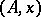, whetherhalts or loops with.

A special case of the halting problem is the self-applicability problem, defined as follows. Every algorithmis completely determined by its Gödel word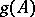. E.g.,can be defined as the list of all instructions in, written one after the other. An algorithmis termed self-applicable exactly in casehalts with. The self-applicability problem consists of deciding whether or not an arbitrary algorithm is self-applicable. The self-applicability problem is a subproblem of the halting problem; consequently, if the former is undecidable, then so is the latter.

Assume that there is an algorithm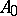for the self-applicability problem. Thus,halts with all inputs of the formand produces the answer "yes" or "no" , depending on whether or notis self-applicable.is now modified by adding a non-terminating computation starting from the "yes" answer. Thus, the modified algorithmloops (respectively, halts) with inputs of the form, whereis (respectively, is not) self-applicable. By diagonalizing, that is, considering the input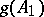for, a contradiction is obtained. This implies that the self-applicability problem is undecidable, and so is the halting problem.

Proofs of algorithmic undecidability are either direct or indirect. A direct proof, such as the one outlined above, normally uses diagonalization in some form. An indirect proof of the undecidability of a problemuses reduction to a problemwhose undecidability is already known: one converts a supposed algorithm for solvinginto an algorithm for solving. Such useful "reference points"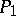among the undecidability problems are Hilbert's tenth problem and the Post correspondence problem.

Decidability is preserved in the transition from a problem to a subproblem of it. Similarly, undecidability is preserved in ascending hierarchies of problems. It is of crucial importance to know the border line between decidability and undecidability. It is very difficult to determine the exact border, but in rougher terms one sometimes succeeds. E.g., consider word problems for groups and semi-groups determined by finitely many defining equations. Consider also unidirectional word problems, where the defining relations allow only the substitution of the right-hand side in place of the left-hand side of the relation, but not vice versa. If also commutativity is considered, eight problems arise in this setup: group versus semi-group, general versus Abelian, and equation versus unidirectional relation. The word problem for groups is undecidable and, hence, so are the three more extensive problems. The unidirectional word problem for Abelian semi-groups is decidable and, hence, so are the three subproblems described above. Thus, in this setup, the border line between decidability and undecidability is known.

For a discussion of propositions which are undecidable within a formal system, see Unsolvability; Gödel incompleteness theorem. It was shown already by K. Gödel that the existence of undecidable propositions is not a shortcoming of any particular formal system, but rather a property inherent in all formal systems. Such an inherently undecidable proposition is the formal statement expressing the consistency of a given formal system. Later it turned out (e.g., due to the work of G. Chaitin [a1], [a2]) that undecidable propositions are far from being rare, but that they are very frequent, often very simple, and that some of them belong to the most elementary arithmetic. It is not anymore possible to disregard undecidable propositions as exceptional singularities that are not encountered in "real mathematics" . As Chaitin puts it: "Non-linear dynamics and quantum mechanics have shown that randomness is present in nature. I believe that I have shown that it is also present in pure mathematics, in fact even in the most elementary branches of the theory of numbers" .

Undecidable statements cannot be ignored. They are not exceptional and pathological but numerous, nearby and palpable. For any formal system, there are genuine arithmetical statements whose truth or falsity cannot be established within the system, although it seems necessary that the statement is either true or false. E.g., such a statement concerns the solvability of a given Diophantine equation. One can construct a sequence of Diophantine equations with many variables, parametrized by, say,. The information contained in the solution of equations corresponding to finitely many values of the parameteris, in general, not applicable in solving the other cases. Mathematical formal reasoning is powerless to link the different cases. Thus, this extra-ordinary parametrized Diophantine equation surpasses what can be achieved by any formal system. No method of solving the different cases is essentially better than putting directly in the axioms the results one wants to obtain!

How to Cite This Entry:
Undecidability. Encyclopedia of Mathematics. URL: http://encyclopediaofmath.org/index.php?title=Undecidability&oldid=12038
This article was adapted from an original article by G. RozenbergA. Salomaa (originator), which appeared in Encyclopedia of Mathematics - ISBN 1402006098. See original article